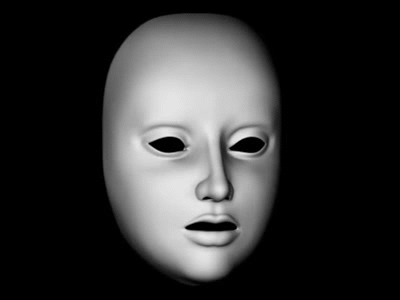October 2020

# Normative Models

## Uncertainty

The world is riddled with uncertainty.NoiseAmbiguityPartial Information

## Probability Theory

Probability theory is nothing but common sense reduced to calculation.

Pierre-Simon Laplace (1819)

## Bayes’ Theorem

Given the state $x$, the observation $y$ and the hypothesis space $\mathcal{H}$

$P(x|y, \mathcal{H}) = \frac{P(y|x, \mathcal{H}) \cdot P(x| \mathcal{H})}{P(y| \mathcal{H})}$

• Likelihood: Probability of the observations given the explanation.
• Prior: Probability of the explanation based on prior experiences.
• Posterior: Probability of the explanation given the observations.

## Posterior Update## Model Selection

What happens when we have multiple explanations $\mathcal{H}_1, \mathcal{H}_2$ for the data? $\mathcal{H}^* = arg \max_{\mathcal{H}_i} P(\mathcal{H}_i|y)$

• Find the most probable explanation by maximisation
• Automatically embodies Occams’ Razor

## Expected Utility Maximisation

$a^* = arg \max_a \int p(x | y) \cdot U(a, x) dy$

• Utility function $U(x, a)$ encodes our own preferences
• Choose the action that maximises utility!

## Ideal-Observer Analysis

Derive a theoretically optimal model on how to perform a given task.## Perceptual Decision Making

In simple perceptual decision tasks, humans perform close to optimal.# Monty Hall Problem# Process Models

## Marr’s Levels of Analysis## Artificial Neural Networks

Study mathematical models of the brain.## Reinforcement Learning

Let artificial agents solve real tasks.// reveal.js plugins